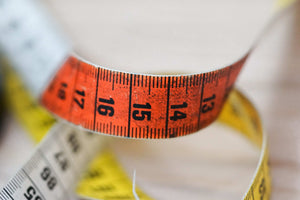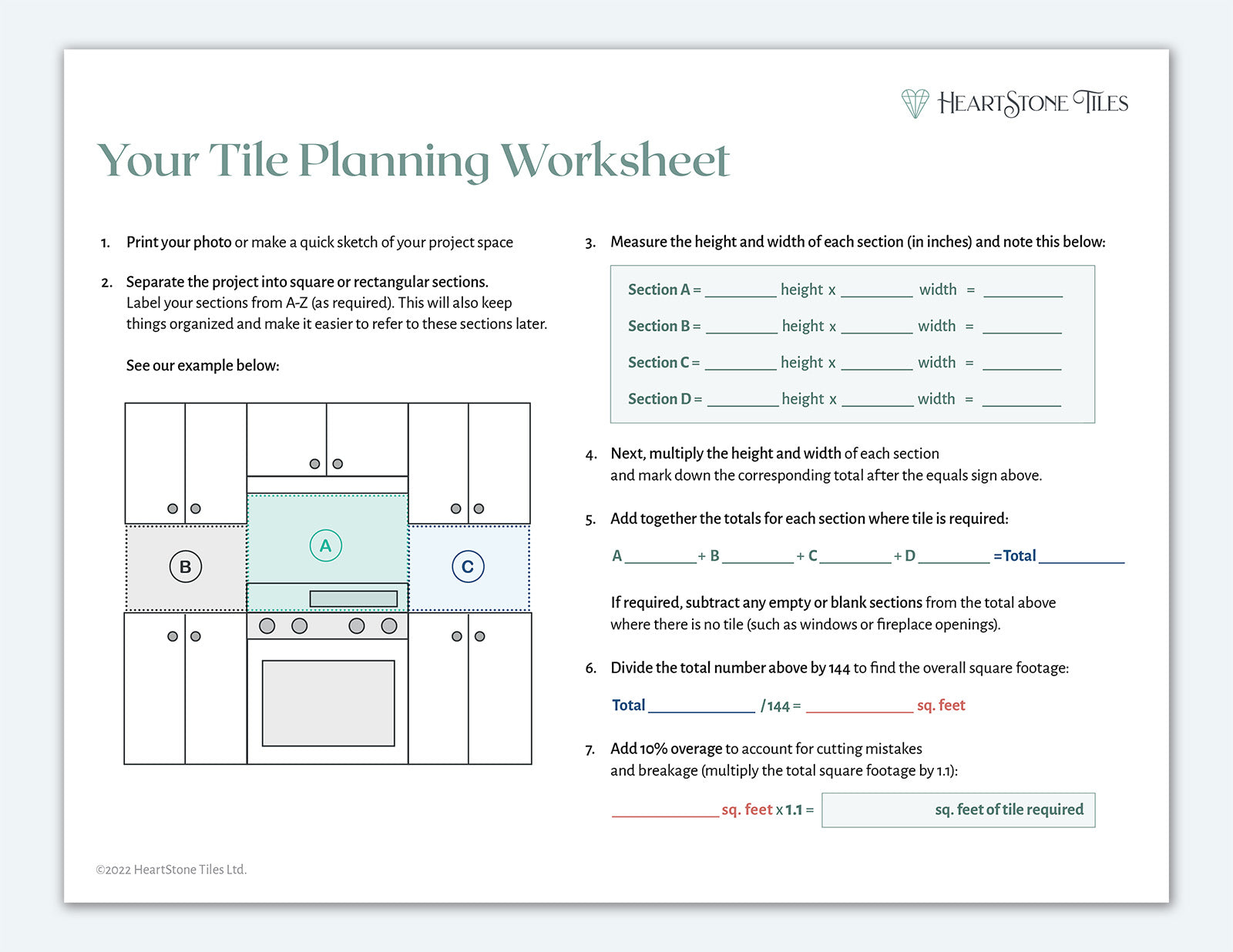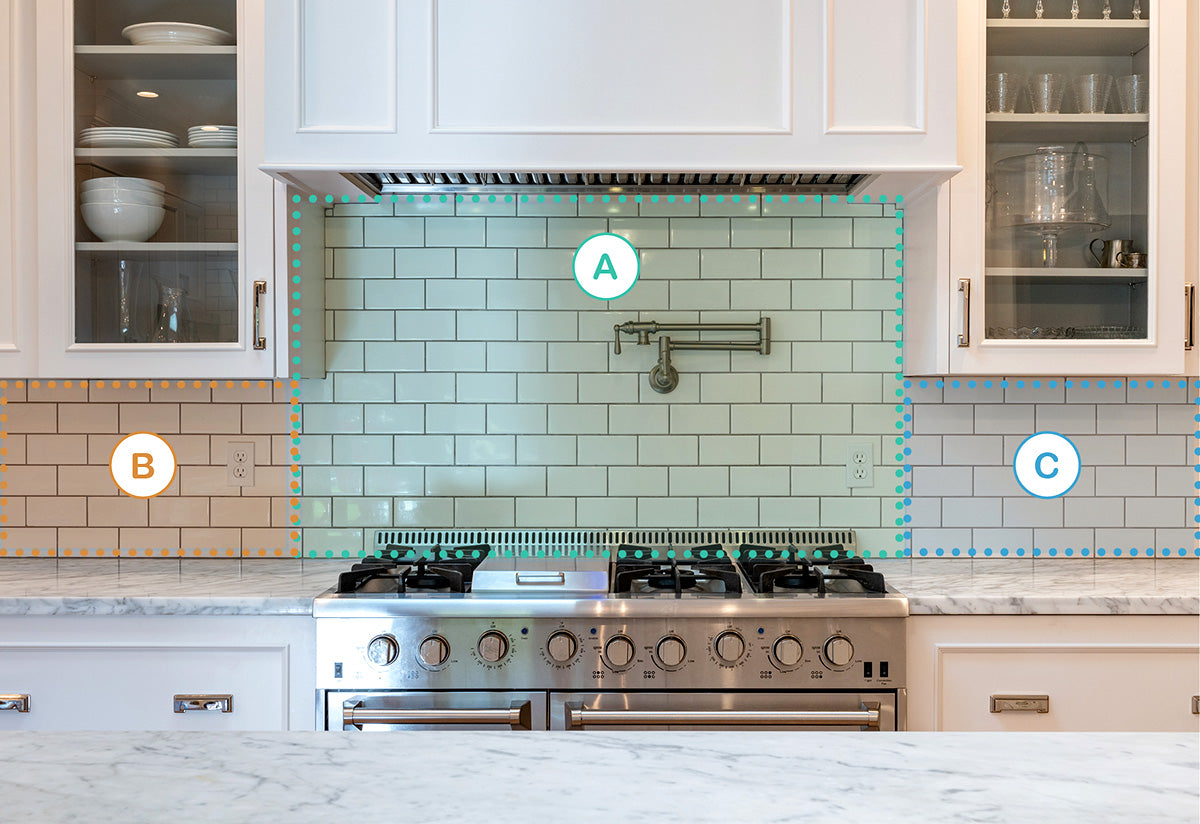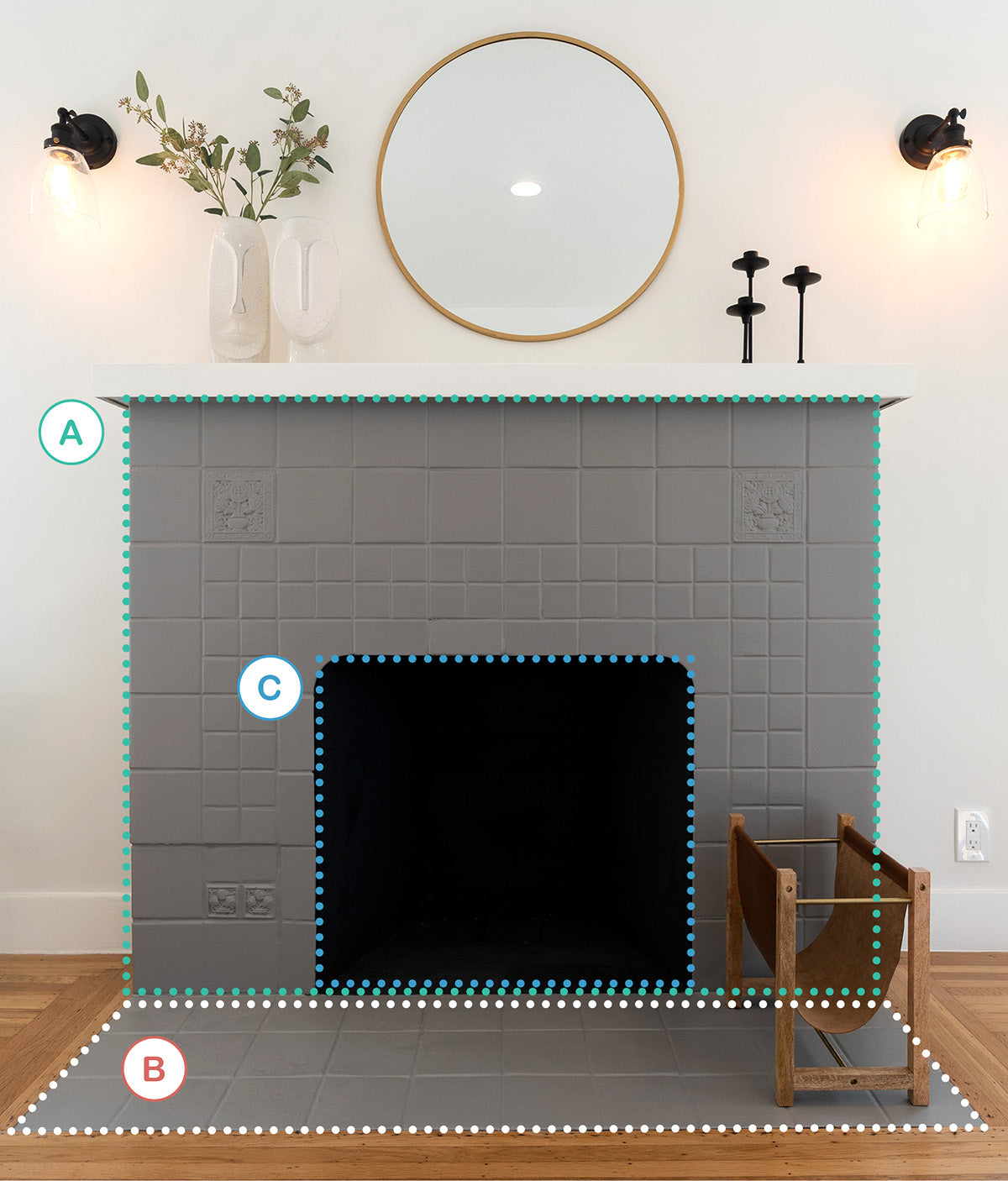# Measuring for Tile – A Helpful Guide for Calculating Tile Square Footage## Trying to determine how much ceramic tile you need for your kitchen backsplash, fireplace, shower wall or floor?

Our easy step-by-step guide will show you how to measure and calculate the square footage of tile that you need – including a helpful worksheet you can download for your next project.

### Step One – Gather Your Measuring and Planning Tools

Most of the tools required for determining how much ceramic tile you need, can be easily found around the home. We recommend the following items:

• Tape measure

• Pencil, eraser and paper

• Calculator

• Photo of the space printed on paper  – straight views are best (or simply make a quick sketch as a reference)

### Step Two – Review Our Examples for Calculating Tile Square Footage

In this article, we will walk you through measuring for tile on both a fireplace and kitchen backsplash tile project.

We will show you how to divide the project into sections to make measuring more easy and how to subtract blank areas such as windows and fireplace openings.

This will help you get a sense of the required steps (it's always best to learn through example).Our Project Planning Guide works like a Tile Calculator by walking you through each step (as shown below) so that you can easily measure your own space and determine the amount of ceramic tile required for your project. Click the image above to download the guide (opens in a new tab).

## Example One – How to Calculate Tile Needed for a Kitchen Backsplash

Step One – Let's start by reviewing the area where we want tile. This project has some irregular areas (such as the higher part of the backsplash above the stove) so we can measure more easily if we break this out into its own section. In this project, we will define three rectangular sections labelled A, B and C.

Draw and label these sections on your printed photo or sketch:Step Two – Next, measure the height and width of each rectangular section (in inches) and label your photo with these measurements.

• Section A is 30 x 46”
• Section B is 15 x 21”
• Section C is 15 x 21”

Step Three – Next, we multiply the height and width of each section and mark the totals down:

• 30 x 46” = 1380
• 15 x 21” = 315
• 15 x 21” = 315

Step Four – Add together the section totals. We don’t need to consider any windows or blank sections in this project.

1380 + 315 + 315 = 2010

Step Five – Divide that number by 144 to find the square footage:

2010 / 144 = 14 sq. feet (rounded up)

Step Six – Add 10% overage to account for cutting mistakes and breakage (we multiply the total square footage by 1.1):

14 sq. feet x 1.1 = 15.4 sq. feet of tile required

## Example Two – How to Determine Tile Needed for a Fireplace Surround

Next, let's learn how to determine the square footage of tile required for this fireplace surround. When tiling a ceramic fireplace surround, you have to account for the firebox opening and subtract this area from your overall calculation.

Step One – We start by reviewing the tile area and separating the project into three rectangular sections labelled A, B and C (mark this down on your printed photo of the fireplace).Step Two – We measure the height and width of each section in inches (including the firebox opening):

• Section A is 52 x 48”
• Section B is 22 x 48”
• Section C is 24 x 22”

Step Three – Next, we multiply the height and width of each section and mark the totals down:

• 52 x 48” = 2496
• 22 x 48” = 1056
• 24 x 22” = 528

Step Four – Then, add together the totals for Sections A and B and subtract the empty area for the firebox opening (Section C):

(2496 + 1056) – 528 = 3024

Step Five – Divide that number by 144 to find the overall square footage:

3024 / 144 = 21 sq. feet

Step Six – Finally, we add 10% overage to account for cutting mistakes and breakage (multiply the total square footage by 1.1):

21 sq. feet x 1.1 = 23.1 sq. feet of tile required